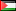##### The Heat Radiation Problem: Three-Dimensional Analysis for Arbitrary Enclosure Geometries
Publication Type
Original research
Authors
• Naji A. Qatanani
• Monika Schulz
Fulltext

This paper gives very significant and up-to-date analytical and numerical results to the three-dimensional heat radiation problem governed by a boundary integral equation. There are two types of enclosure geometries to be considered: convex and nonconvex geometries. The properties of the integral operator of the radiosity equation have been thoroughly investigated and presented. The application of the Banach fixed point theorem proves the existence and the uniqueness of the solution of the radiosity equation. For a nonconvex enclosure geometries, the visibility function must be taken into account. For the numerical treatment of the radiosity equation, we use the boundary element method based on the Galerkin discretization scheme. As a numerical example, we implement the conjugate gradient algorithm with preconditioning to compute the outgoing flux for a three-dimensional nonconvex geometry. This has turned out to be the most efficient method to solve this type of problems.

Journal
Title
Journal of Applied Mathematics Volume 2004 (2004), Issue 4, Pages 311-330
Publisher
--
Publisher Country
PalestinePublication Type
Both (Printed and Online)
Volume
--
Year
2004
Pages
--